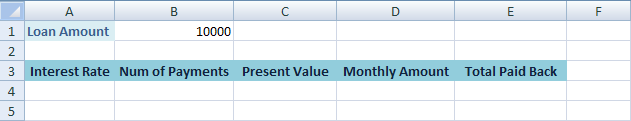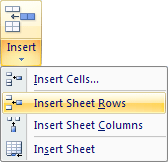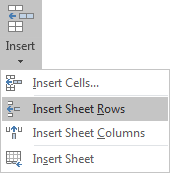Home and Learn: Microsoft Excel Course

# Financial Functions in Excel

The financial function we're going to explore is called PMT( ). You use this function when you want to calculate things like the monthly payment amounts on a loan, or how much per month a mortgage will cost you. We'll use it to work out how much per month a loan will cost us. Here's what we'll do.

We've decided to take out a loan of ten thousand pounds from our friendly banker. We're going to be paying it back over 5 years. The question is, how much per month is this going to cost us?

### The PMT( ) Function in Excel

The PMT( ) function expects certain values in between its two round brackets. The values that go in round brackets are known as arguments. The arguments for the PMT( ) function are these:

PMT(rate, nper, pv, fv, type)

Only the first three are needed, and you can miss the final two out, if you like.

We'll work out our monthly loan costs with the help of the PMT( ) function. First, create a new spreadsheet like the one below:If you look at cell B1 on the spreadsheet, you'll see a figure of £10, 000. This is the amount we want to borrow. The labels on Row 3 show what else we need: An interest rate, the number of payments we'll make over the 5 years, the present value of the loan, the amount we'll have to pay back each month, and the total amount paid back after 5 years. But we only need the first three for our PMT() function.

In cell A4, we'll need an interest rate. In cell B4 we'll need the number of payments, and in cell D4 we'll need the Present Value of the loan. First is interest rate.

Imagine that the interest rate given to us by the bank is 24 percent per year. For the PMT( ) function, we need to divide this figure by 12 (the number of months in a year) So try this:

• Enter the following formula:
= 24% / 12
• Hit the enter key to see the answer appear, as in the image below:Now that we have an interest rate, the next thing we need for the PMT( ) function is how many payments there are in total. We have to pay something back every month for 5 years. Which is a simple formula. So,

= 12 * 5

• Hit the enter key to see a figure of 60 as the answer.

This figure of 60 is for the second argument of the PMT( ) function - the nper. This is just the number of payments.

Now that you have a figure in cell A4 (rate), and a figure in cell B4 (nper), there's only one more to go - the Present Value (pv).

The Present Value of a loan, also known as the Principal, is what the loan is worth at the present time. Since we haven't made any payments yet, this is just 10, 000 for us.

• Click into cell C4 on your spreadsheet and enter the following:

= B1

• Hit the enter key
• You'll see a figure of 10, 000 appear, and your spreadsheet should now look ours below:OK, we now have all the parts for our PMT() function: a rate (A4), an nper (B4), and a pv (C4). Try this:

• Enter the following function:

=PMT(A4, B4, C4)

Hit the enter key on your keyboard, and you'll see the monthly amount appear. The figure you should have is -£287.68. The reason there is a minus sign before the total is because it's a debt: what you owe to the bank.The only thing left to do is see how much this loan will cost us at the end of 5 years. All you need to do here is multiply the monthly amount in cell D4 by the number of payments in cell B4. Enter your formula for this in cell E4, and you spreadsheet will look like ours below:So a ten thousand pounds loan, at the interest rate the bank is offering, means we'll have to pay back just over 17 thousand pounds over 5 years.

### Tweaking the Values

We can change the spreadsheet slightly to give us more control. For your figure in cell B4, the number of payments, you entered 12 * 5. This is 12 months multiplied by 5 years. But what if we wanted to pay the loan back over 10 years, or 15? How much will our monthly payments then be? And will be the final cost of the loan?

Also, the interest rate seems a bit high. What if we can get a better rate elsewhere?

By making a few changes to or spreadsheet, we can amend these values more easily. First we'll need two new rows.

### Inserting New Rows in Excel

We need to insert new rows in our spreadsheet. To insert a new row, click into cell A2. Then click on the Home tab at the top of Excel. Locate the Cells panel, and click the Insert item:Insert Sheet Rows:Num of Years and Interest. Your spreadsheet sheet will then look like this:We can adapt the formulas we've entered so far, in order to make them more usable. As an example, we'll adapt the interest rate.

To get the interest rate for cell A4, we entered a formula:

= 24% / 12

Instead of having the interest rate in cell A4, however, we can place it at the top, in cell B3 on our new Row. We can then alter the interest rate by simply typing a new one in cell B3. To clear all that up, try the following:

• Click inside cell B3, which is the Interest cell in the image above
• Click inside the formula bar
• Type in = 24%
• Cell B3 should now read 24.00% (In Excel 2010 and 2013 you may have to format the cell to a Percentage value from the Home tab then the Numer item.)
To change the formula for your interest rate click inside of cell A6. Change the formula from this:

= 24% / 12

to this:

= B3 / 12

Hit the enter key on your keyboard and nothing should change on your spreadsheet. But the difference is that you can enter a new interest rate in cell B3, and see how this effects the loan amounts. Try it out by typing 23% in cell B3:As you can see, the interest rate has changed to a rather long figure. But notice the Monthly Amount - it has gone down to £281.90. The total amount we have to pay back has changed, too. Play around with the interest rate in cell B3, just to get a feel for how it works.

Exercise

= 12 * 5

This calculates the number of months for the loan. Change this formula so that the number of years is coming from B2. Your finished spreadsheet should look like ours below:If you play around with the values in cells B1, B2 and B3 you should be able quickly see the new loan repayments.

In the next part, you'll see what Conditonal Logic is, and how to use it in Excel. First, try this project. It's all to do with Averages, so shouldn't cause you too many problems.

<--Back to the Excel Contents Page

Email us: enquiry at homeandlearn.co.uk